# Homogoraphy: A Case Study in Model Fitting¶

Faisal Qureshi
Professor
Faculty of Science
Ontario Tech University
http://vclab.science.ontariotechu.ca

## Homography¶

Projection of a point in a world plane to the image plane. The location of the point and that of its projection is related via homography.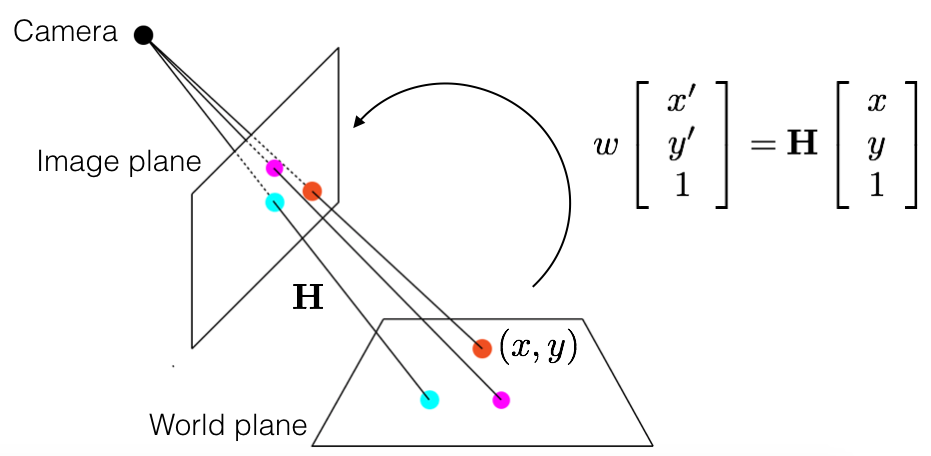Generally speaking, points that lie on two planes are related via homography. This also means that the projections of points (that lie on a common plane) in two cameras are related via homography.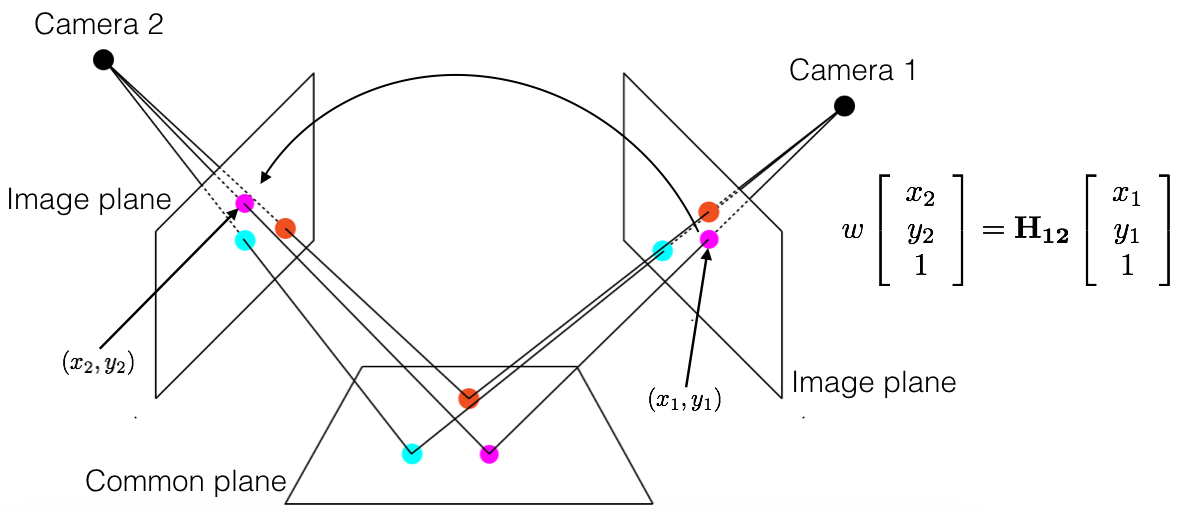## Image Stitching¶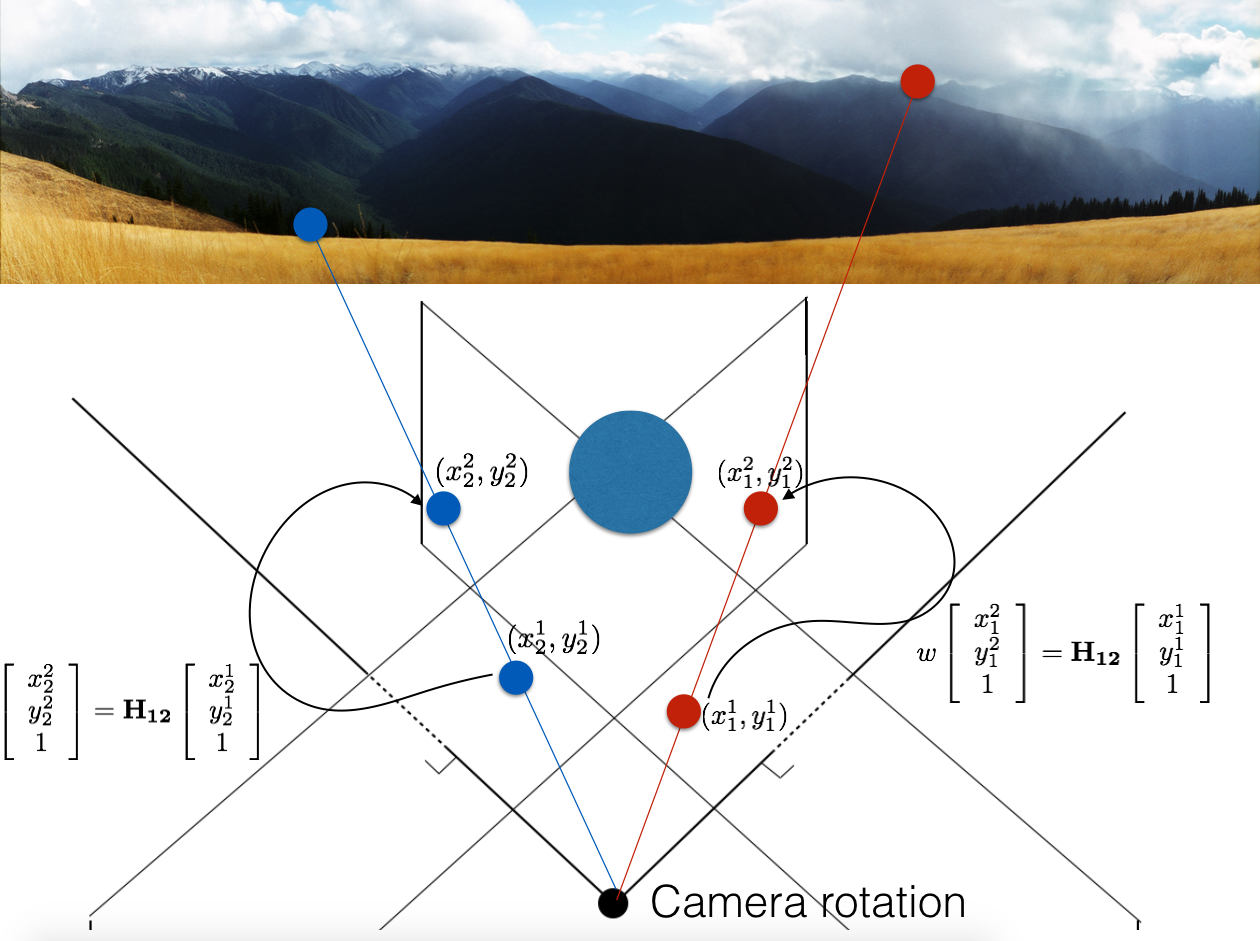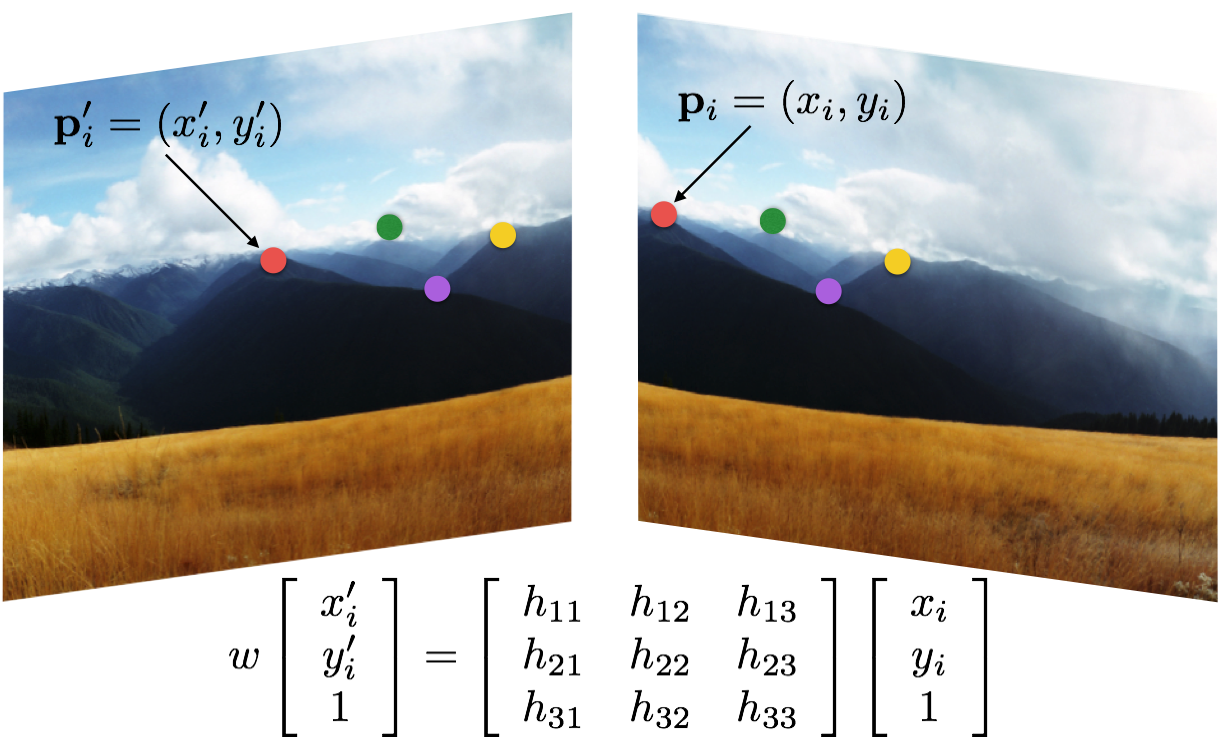## Exercise 1¶

How many correspondences do we need to solve $\mathbf{H}$, where

$$\mathbf{H} = \left[ \begin{array}{ccc} h_{11} & h_{12} & h_{13} \\ h_{21} & h_{22} & h_{23} \\ h_{31} & h_{32} & h_{33} \end{array} \right].$$

### Solution Exercise 1¶

This matrix can only be known upto a scale, so it has 8 degrees of freedom. We need at least 4 correspondences to solve this matrix.

This matrix often cannot be solved using pseudo-inverse technique due to numerical issues.

## Solving for Homography¶

Re-write homography relationship as homogeneous equations

\begin{align} x'_i &= \frac{h_{11} x_i + h_{12} y_i + h_{13}}{h_{31} x_i + h_{32} y_i + h_{33}} \\ y'_i &= \frac{h_{21} x_i + h_{22} y_i + h_{23}}{h_{31} x_i + h_{32} y_i + h_{33}} \end{align}

We can then write these as matrix-vector product

$$\left[ \begin{array}{ccccccccc} x_i & y_i & 1 & 0 & 0 & 0 & -x_i x'_i & -y_i x'_i & - x'_i \\ 0 & 0 & 0 & x_i & y_i & 1 & -x_i y'_i & -y_i y'_i & - y'_i \end{array} \right] \left( \begin{array}{c} h_{11} \\ h_{12} \\ h_{13} \\ h_{21} \\ h_{22} \\ h_{23} \\ h_{31} \\ h_{32} \\ h_{33} \end{array} \right) = \mathbf{0}$$

Given $n$ point correspondences between two images, we set up $mathbf{A} \mathbf{x} = 0$ as follows:

\begin{align} \left[ \begin{array}{ccccccccc} x_1 & y_1 & 1 & 0 & 0 & 0 & -x_1 x'_1 & -y_1 x'_1 & - x'_1 \\ 0 & 0 & 0 & x_1 & y_1 & 1 & -x_1 y'_1 & -y_1 y'_1 & - y'_1 \\ &&&&& \vdots &&& \\ x_i & y_i & 1 & 0 & 0 & 0 & -x_i x'_i & -y_i x'_i & - x'_i \\ 0 & 0 & 0 & x_i & y_i & 1 & -x_i y'_i & -y_i y'_i & - y'_i \\ &&&&& \vdots &&& \\ x_n & y_n & 1 & 0 & 0 & 0 & -x_n x'_n & -y_n x'_n & - x'_n \\ 0 & 0 & 0 & x_n & y_n & 1 & -x_n y'_n & -y_n y'_n & - y'_n \end{array} \right] \left( \begin{array}{c} h_{11} \\ h_{12} \\ h_{13} \\ h_{21} \\ h_{22} \\ h_{23} \\ h_{31} \\ h_{32} \\ h_{33} \end{array} \right) &= \mathbf{0} \\ \mathbf{A} \mathbf{x} & = \mathbf{0} \end{align}

$\mathbf{x}$ is the vector of unknowns.

### Estimating $\mathbf{x}$¶

• Estimate using least-square fitting $$\mathbf{x}^* = \underset{x}{\operatorname{argmax}} \| \mathbf{A} \mathbf{x} \|^2\ \mathrm{s.t.}\ \| \mathbf{x} \|=1$$
• Note that the solution is also the right null-space of $\mathbf{A}$, so the solution is the eigenvector corresponding to the smallest eigenvalue of $\mathbf{A}^T \mathbf{A}$.

## Image Stitching¶

• Estimate homography
• Use it to fill in the colors from the "other" image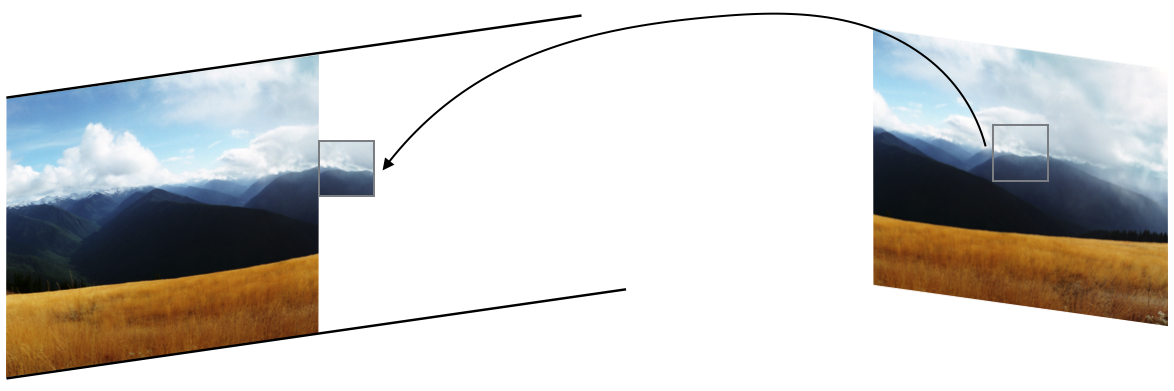### Image Stitching - Steps¶

Extract features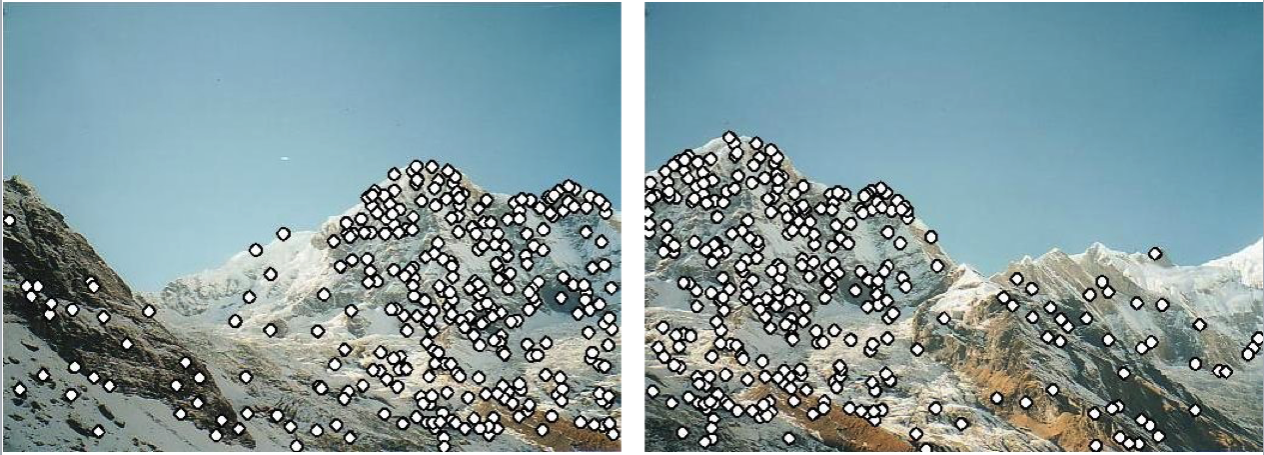Find matches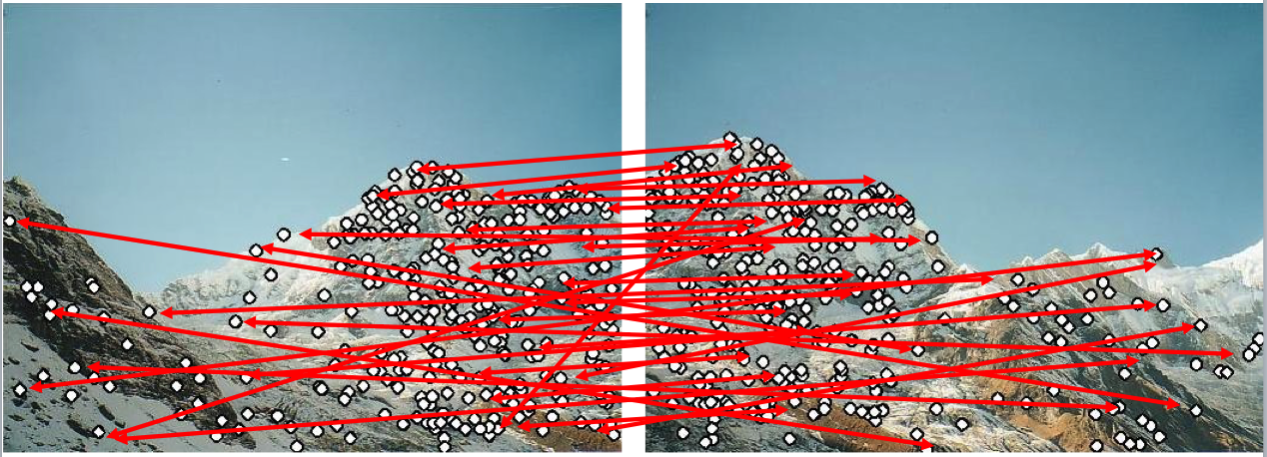Use RANSAC to estimate homography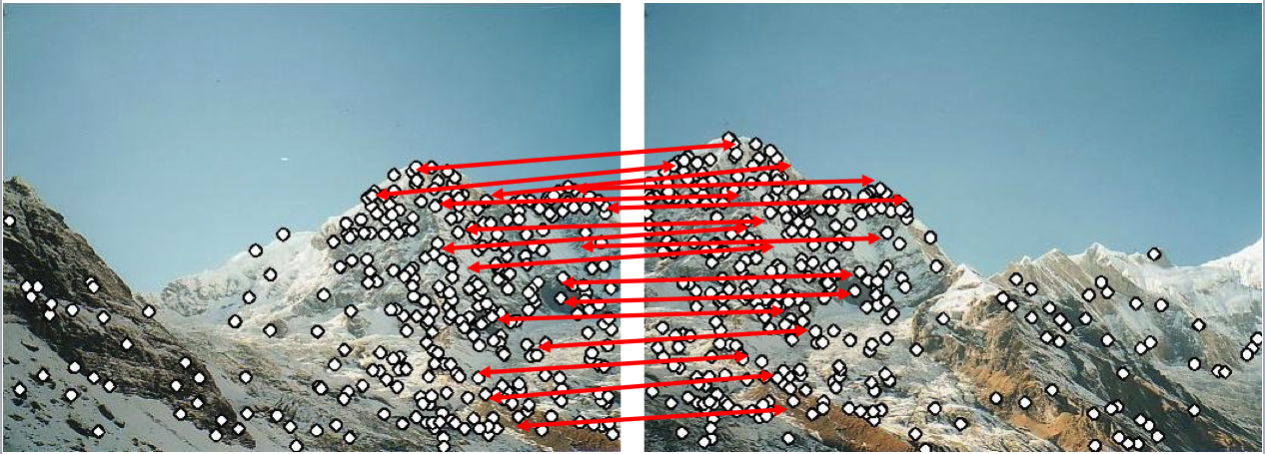Perform image stitching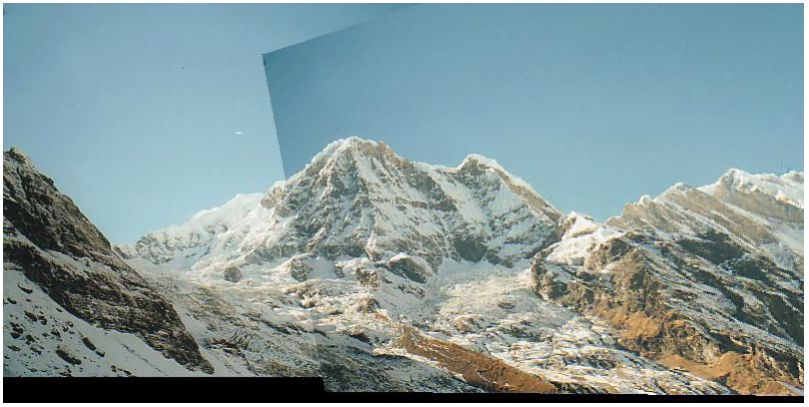In [ ]: